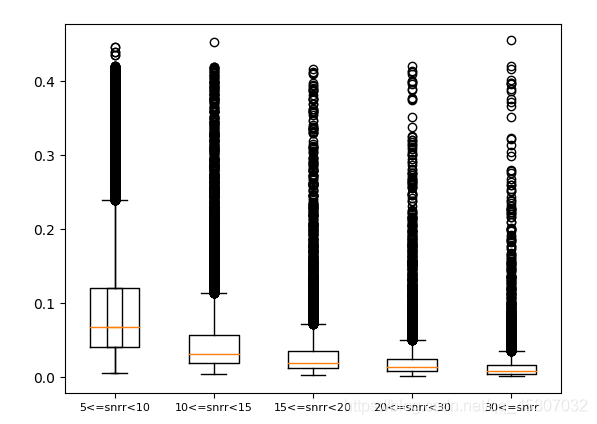# Python使用plt.boxplot()函數繪制箱圖、常用方法以及含義詳解

## 1. 箱圖含義## 2.計算方法

Q1：中位數將所有數據分成兩部分，最小值到中位數的部分按取中位數的方法取中位數作為Q1。

Q3：同Q1取法，取中位數到最大值的中位數。

IQR(四分位數間距)=Q3-Q1

## 3.繪圖

### 3.1 繪制單個箱圖

```import matplotlib.pyplot as plt
import numpy as np

#生成data數據
np.random.seed(100)
data = np.random.normal(size=(1000,),loc=0,scale=1)

# 繪圖
plt.boxplot(data)
plt.show()
```### 3.2 繪制多個箱圖

```import matplotlib.pyplot as plt
import numpy as np

np.random.seed(100)
data = np.random.normal(size=(1000,4),loc=0,scale=1)

plt.boxplot(data)

plt.show()
```### 3.3實戰

```def plt_box_iamge(df):
"""
snrr的五個范圍為[5,10)、[10,15)、[15,20)、[20,30)、[30-)，按照五個snrr范圍計算對應redchi的箱圖
:param df:包含snrr以及redchi的csv數據（dataFrame）。
:return:
"""
# 根據snrr范圍對redchi進行篩選。
df1 = df.loc[df['lam_snrr'] >= 5]
redchi_1 = df1.loc[df1['lam_snrr'] < 10].redchi

df2 = df.loc[df['lam_snrr'] >= 10]
redchi_2 = df2.loc[df2['lam_snrr'] < 15].redchi

df3 = df.loc[df['lam_snrr'] >= 15]
redchi_3 = df3.loc[df3['lam_snrr'] < 20].redchi

df4 = df.loc[df['lam_snrr'] >= 20]
redchi_4 = df4.loc[df4['lam_snrr'] < 30].redchi

redchi_5 = df.loc[df['lam_snrr'] >= 30].redchi
# 繪圖
ax = plt.subplot()
ax.boxplot([redchi_1, redchi_2, redchi_3, redchi_4, redchi_5])
# 設置軸坐標值刻度的標簽
ax.set_xticklabels(['5<=snrr<10', '10<=snrr<15', '15<=snrr<20', '20<=snrr<30', '30<=snrr'], fontsize=8)
#	保存圖片
plt.savefig('./images/box.jpg')
plt.show()

if __name__ == '__main__':
df_sc = screening(df)  # 篩選數據 (lamost數據應該在正常值范圍內，不然因為數值差過大會導致繪制不出圖像!)
plt_box_iamge(df_sc)
```### 3.3 參數詳解

```plt.boxplot(x,                      # x：指定要繪制箱圖的數據
notch=None,           # notch：是否是凹口的形式展現箱線圖，默認非凹口
sym=None,              # sym：指定異常點的形狀，默認為+號顯示
vert=None,              # vert：是否需要將箱線圖垂直擺放，默認垂直擺放
whis=None,             # whis：指定上下須與上下四分位的距離，默認為1.5倍的四分位差
positions=None,   # positions：指定箱線圖的位置，默認為[0,1,2…]
widths=None,         # widths：指定箱線圖的寬度，默認為0.5
patch_artist=None,        # patch_artist：是否填充箱體的顏色
meanline=None,             # meanline：是否用線的形式表示均值，默認用點來表示
showmeans=None,       # showmeans：是否顯示均值，默認不顯示
showcaps=None,           # showcaps：是否顯示箱線圖頂端和末端的兩條線，默認顯示
showbox=None,             # showbox：是否顯示箱線圖的箱體，默認顯示
showfliers=None,          # showfliers：是否顯示異常值，默認顯示
boxprops=None,           # boxprops：設置箱體的屬性，如邊框色，填充色等
labels=None,                  # labels：為箱線圖添加標簽，類似於圖例的作用
flierprops=None,          # filerprops：設置異常值的屬性，如異常點的形狀、大小、填充色等
medianprops=None,   # medianprops：設置中位數的屬性，如線的類型、粗細等
meanprops=None,       # meanprops：設置均值的屬性，如點的大小、顏色等
capprops=None,           # capprops：設置箱線圖頂端和末端線條的屬性，如顏色、粗細等
whiskerprops=None)   # whiskerprops：設置須的屬性，如顏色、粗細、線的類型等
```

### 3.4 常用方法

```import matplotlib.pyplot as plt
import numpy as np

np.random.seed(100)
data = np.random.normal(size=(1000,4),loc=0,scale=1)

ax = plt.subplot()
ax.boxplot(data)                                 # 繪圖
ax.set_xlim([0,5])                               # 設置x軸值的范圍  rotation=30
# ax.set_xticks()  							      # 自定義x軸的值
ax.set_xlabel("xlabel")                  # 設置x軸的標簽
ax.set_xticklabels(['A','B','C','D'],  rotation=30,fontsize=10)   # 設置x軸坐標值的標簽 旋轉角度 字體大小
ax.set_title("xcy")       					  # 設置圖像標題
ax.legend(labels= ['A','B','C','D'],loc='best',)  # 增加圖例
ax.text(x=0.2 , y=3.5 , s="test" ,fontsize=12)   # 增加註

plt.show()```

matplotlib官方文檔